Surface Brightness ProfileNext: Velocity Field Up: Fitting Model Previous: Parameters

Surface Brightness Profile

The synthetic rotation curves used a thin exponential disk model for the [OII] surface brightness distribution. In this model, the surface brightness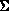as a function of radius from the center of a galaxy is given by the simple equation: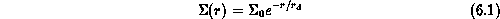where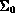and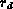are the central surface brightness and scale length of the disk respectively. Equationprovides a good fit to the light profiles of spiral disk galaxies. The central surface brightnessof an exponential disk is related to the total luminosity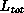of the disk by: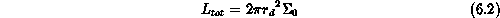Equationis obtained by simply integrating equationfrom zero to infinity. In real disk galaxies, surface brightness profiles are influenced by complex internal absorption by dust lanes. Internal absorption by dust lanes was not taken into account in the fitting model since dust distributions vary from galaxy to galaxy, and they can only be mapped with HST's high spatial resolution at intermediate redshifts. Every point in the simulated disk was given an observed intensity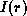following equation.

Luc Simard
Mon Sep 2 12:37:40 PDT 1996